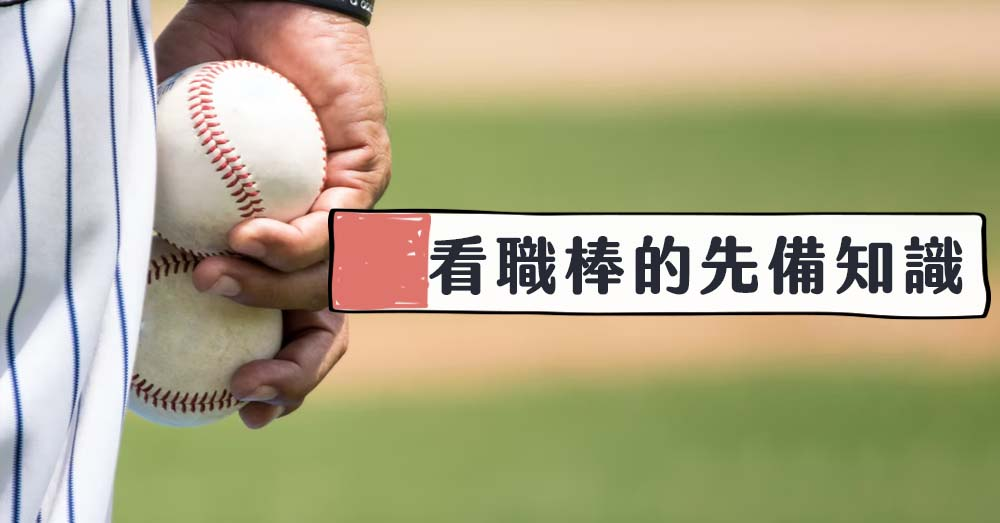# 全壘打、安打、三振等棒球術語日文怎麼說?### ・看板標記

 標記 B S O 日文 ボール ストライク アウト 中文 壞球 好球 出局

### ・基礎、進階詞彙＆裁判用語

 日文 中文 日文 中文 ヒット 安打 シングルヒット 単(たん)打(だ) 一壘安打 ツーベースヒット 二(に)塁(るい)打(だ) 二壘安打 スリーベースヒット 三(さん)塁(るい)打(だ) 三壘安打 ホームラン 本(ほん)塁(るい)打(だ)、 一(いっ)発(ぱつ) 全壘打 サヨナラホームラン 再見全壘打 ソロホームラン 陽春全壘打 空(から)振(ぶ)り 揮棒落空 三(さん)振(しん) 三振 四(し)球(きゅう) 四壞球保送 ダブルプレイ 雙殺打 トリプルプレイ 三殺打 タッチアウト 觸殺 ファウルボール 界外球 フェアボール 界內球 デッドボール 觸身球 ゴロ 滾地球 フライ 高飛球 バント 短打 セーブ 安全上壘 満(まん)塁(るい) 滿壘 スチール 盗(とう)塁(るい) 盜壘 スローイング 傳球 スイング 揮棒 エラー 失誤 タイム 暫停 打(だ)順(じゅん) 棒次 背(せ)番(ばん)号(ごう) 球衣背後號碼 攻(こう)撃(げき)側(がわ) 進攻隊伍 守(しゅ)備(び)側(がわ) 防守隊伍 表(おもて) 上半局 裏(うら) 下半局 点(てん)差(さ) 得分差距 同(どう)点(てん) 同分 勝(か)ち越(こ)し 領先 逆(ぎゃく)転(てん) 逆轉 ピンチヒッター 代(だい)打(だ) 代打 指(し)名(めい)打(だ)者(しゃ) 指定打擊 空(から)振(ぶ)り三(さん)振(しん) 揮棒落空被三振 見(み)逃(のが)し三(さん)振(しん) 站著被三振 振(ぶ)り逃(に)げ 不死三振 犠(ぎ)打(だ) 犧牲打 送(おく)りバント、 犠(ぎ)牲(せい)バント 犧牲觸擊 スクイズ 強迫取分 サイクルヒット 一(いち)巡(じゅん)安(あん)打(だ) 完全打擊 打(だ)率(りつ) 打擊率

### ・守備＆攻擊位置、角色名稱

 日文 中文 日文 中文 バッター 安(あん)打(だ)（あんだ） 打者 ピッチャー 投(とう)手(しゅ) 投手 キャッチャー 捕(ほ)手(しゅ) 捕手 內(ない)野(や)手(しゅ) 內野手 外(がい)野(や)手(しゅ) 外野手 レフト 左(さ)翼(よく)手(しゅ) 左外野手 センター 中(ちゅう)堅(けん)手(しゅ) 中外野手 ライト 右(う)翼(よく)手(しゅ) 右外野手 ファースト 一(いち)塁(るい)手(しゅ) 一壘手 セカンド 二(に)塁(るい)手(しゅ) 二壘手 サード 三(さん)塁(るい)手(しゅ) 三壘手 ショート 遊(ゆう)撃(げき)手(しゅ) 游擊手 コーチ 監(かん)督(とく) 教練 審(しん)判(ぱん) 裁判 ルーキー 新(しん)人(じん) 新人球員 エース 王牌球員

### ・技巧＆招式名稱

 日文 中文 ストレート、速(そっ)球(きゅう)、真(ま)っ直(す)ぐ、速(はや)い球(たま)、直(ちょっ)球(きゅう)、ファストボール 直球 カーブ、緩(ゆる)い球(たま)、遅(おそ)い球(たま) 曲球 フォーク、落(お)ちる球(たま) 指叉球 変(へん)化(か)球(きゅう) 變化球 スライダー 滑球 チェンジアップ 變速球 シンカー 伸卡球

### ・職棒比賽地點名稱

 日文 中文 東(とう)京(きょう)ドーム 東京巨蛋 明(めい)治(じ)神(じん)宮(ぐう)野(や)球(きゅう)場(じょう) 明治神宮棒球場 千(ち)葉(ば)マリンスタジアム 千葉ZOZO海洋球場 阪(はん)神(しん)甲(こう)子(し)園(えん)球(きゅう)場(じょう) 阪神甲子園棒球場 福(ふく)岡(おか)ドーム 福岡巨蛋

#### 重點單字整理